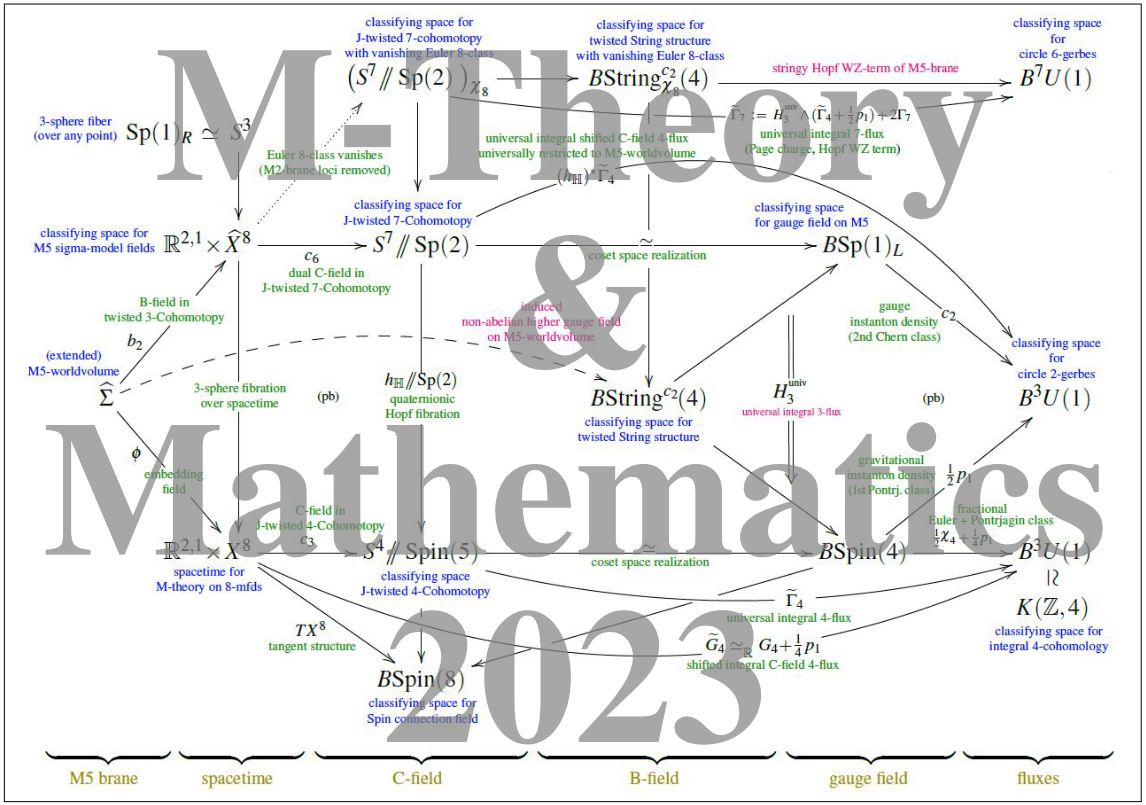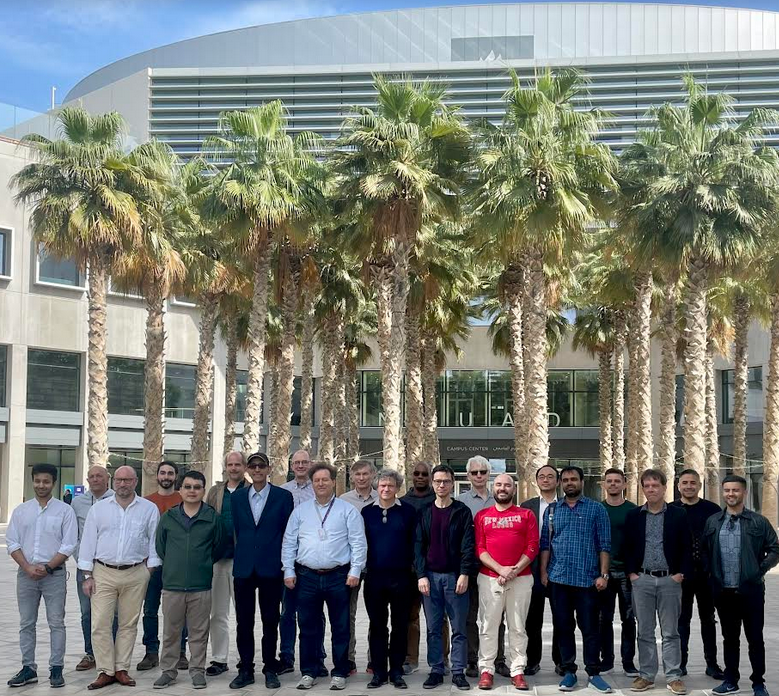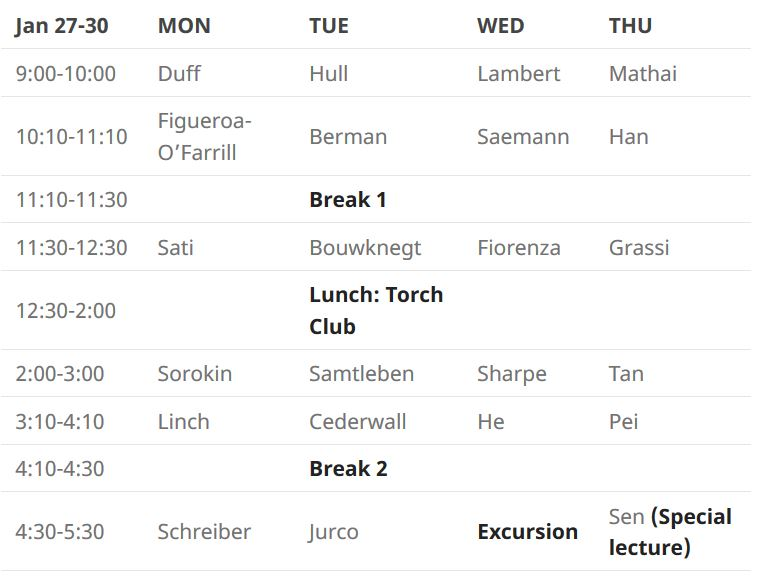# nLab M-Theory and Mathematics

## Phenomenology

#### Quantum systems

A conference series on M-theory and mathematics.

These workshops bring together experts on the mathematical aspects of M-theory, the main candidate for a theory of everything, with implications to string theory and quantum field theory and interactions with geometry and algebraic topology.

# Contents

## M-Theory and Mathematics 2023

• on: January 12-15, 2023

at: CQTS @ New York University, Abu Dhabi

see: event webpage$\phantom{-----}$ (logo adapted from JMP 62 (2021) 042301)

Speakers:(from left to right: Valera, Jurčo, Berman, Alfonsi, Han, Schreiber, Mathai, Saemann, Sati, Voronov, Lambert, Bah, Tsimpis, Hull, Marrani, He, Singh, Giotopoulos, Szabo, )

Titles, Abstracts, and Slides for M-Theory & Math 2023 (in chronological order of talks delivered):

• Universal accelerating cosmologies from 10d supergravity

slides: pdf

video: YT

cf.: arXiv:2210.10813

4d Friedmann-Lemaître-Robertson-Walker cosmologies are obtained from time-dependent compactifications of Type IIA 10d supergravity on various classes of 6d manifolds. These cosmologies are universal in that they do not depend on the detailed features of the compactification manifold, but only on the properties which are common to all the manifolds belonging to that class. Once the equations of motion are rewritten as an appropriate dynamical system, the existence of solutions featuring a phase of accelerated expansion is made manifest. Some of the resulting cosmologies exhibit eternal or semi-eternal acceleration, whereas others allow for a parametric control on the number of $\mathrm{e}$-foldings. Moreover, we find several smooth accelerating cosmologies without Big Bang singularities: the universe is contracting in the cosmological past ($T \lt 0$), expanding in the future ($T \gt 0$), while in the vicinity of $T = 0$ it becomes de Sitter in hyperbolic slicing. We also obtain several cosmologies featuring an infinite number of cycles of alternating periods of accelerated and decelerated expansions.

• Graded T-duality with H-flux for 2d sigma models

slides: pdf

cf.: arXiv:2207.03134

T-duality in string theory can be realised as a transformation acting on the worldsheet fields in the two-dimensional nonlinear sigma model. Bouwknegt-Evslin-Mathai established the T-duality in a background flux for the first time upon compactifying spacetime in one direction to a principal circle by constructing the T-dual maps transforming the twisted cohomology of the dual spacetimes. In this talk, we will describe our recent work on how to promote the T-duality maps of Bouwknegt-Evslin-Mathai in two aspects. More precisely, we will introduce (1) graded T-duality, concerning the graded T-duality maps of all levels of twistings; (2) the 2-dimensional sigma model picture, concerning the double loop space of spacetimes. This represents our joint work with Mathai.

• An introduction to decomposition

slides: pdf

video: YT

cf.: arXiv:2204.09117

In this talk I will review work on ‘decomposition’, a property of 2d theories with 1-form symmetries and, more generally, d-dim’l theories with $(d-1)$-form symmetries. Decomposition is the observation that such quantum field theories are equivalent to (‘decompose into’) disjoint unions of other QFTs, known in this context as “universes”.

Examples include two-dimensional gauge theories and orbifolds with matter invariant under a subgroup of the gauge group. Decomposition explains and relates several physical properties of these theories – for example, restrictions on allowed instantons arise as a “multiverse interference effect” between contributions from constituent universes.

First worked out in 2006 as part of efforts to understand string propagation on stacks, decomposition has been the driver of a number of developments since. I will walk through general aspects of 2d gauge theories that exhibit decomposition, dive into specifics in orbifold examples, and as time permits, discuss recent work in progress.

• Matrix Quantization of Classical Nambu Brackets and Super $p$-Branes

video: YT

cf.: arXiv:2103.06666

We will present an explicit matrix algebra quantization of the algebra of volume-preserving diffeomorphisms of the $n$-torus. That is, we approximate the corresponding classical Nambu brackets using $\mathfrak{sl}\big(N^{\lceil n/2\rceil}, \mathbb{C}\big)$-matrices equipped with the finite bracket given by the completely anti-symmetrized matrix product, such that the classical brackets are retrieved in the $N \to \infty$ limit. We then apply this approximation to the super 4-brane in 9 dimensions and give a regularized action in analogy with the matrix quantization of the supermembrane. This action exhibits a reduced gauge symmetry that we discuss from the viewpoint of $L_\infty$-algebras in a slight generalization to the construction of Lie 2-algebras from Bagger-Lambert 3-algebras.

• Exceptional super Yang-Mills in $27 + 3$ and worldvolume M-theory

slides: pdf

video: YT

cf.: arXiv:1906.10709

Some time ago, Bars and Sezgin proposed a super Yang-Mills theory in $D=s+t=11+3$ space-time dimensions, with an electric 3-brane that generalizes the 2-brane of M-theory. More recently, Rios, Chester and the speaker found an infinite family of “exceptional” super Yang-Mills theories in $D=s+t=(8n+3)+3$. A particularly interesting case occurs for $n=3$, namely in signature $D=27+3$, in which the superalgebra is centrally extended by an electric 11-brane and its 15-brane magnetic dual. The worldvolume symmetry of the 11-brane has signature $D=11+3$ and can reproduce super Yang-Mills theory in D=$11+3$. Upon reduction to $D=26+2$, the 11-brane reduces to a 10-brane with $10+2$ worldvolume signature. A single time projection gives a $10+1$ worldvolume signature, and it can serve as a model for $D=10+1$ M-theory as a reduction from the $D=26+1$ signature of the bosonic M-theory of Horowitz and Susskind; this is further confirmed by the reduction of chiral $(1,0)$, $D=11+3$ superalgebra to the $\mathcal{N}=1$ superalgebra in $D=10+1$, as found by Rudychev, Sezgin and Sundell some time ago. Extending previous results of Dijkgraaf, Verlinde and Verlinde, I will also put forward the realization of spinors as total cohomologies of (the largest spatially extended) branes which centrally extend the $(1,0)$ superalgebra underlying the corresponding “exceptional” super Yang-Mills theory. Moreover, by making use of an “anomalous” Dynkin embedding, I will present further evidence about Ramond‘s and Sati’s argument that M-theory has hidden Cayley plane fibers.

## M-Theory and Mathematics 2020Confirmed speakers:

Abstracts, Slides and Talk notes:

• M-theory and cohomotopy

slides: pdf

Cohomotopy theory has recently emerged as the proper generalized cohomology theory to describe the fields in M-theory. It is hoped that viewing M-theory from a mathematical perspective will shed the light on the nature of the theory and will allow for progress. I will survey this area, illustrating how cohomotopy captures the nature of the C-field and its dual as well as of the M-branes, allows for cancellation of various anomalies, and provides a firm grounding for further study of M-theory. This is joint work Urs Schreiber and Domenico Fiorenza.

This is joint work with H. Sati (arxiv:1909.12277, arxiv:1912.10425).

• Exceptional Field Theories and AdS Compactifications

video: YT

• Bianca Cerchiai

Supergravity in a pencil

slides: pdf slides

video: YT

In the spirit of the gauge-gravity correspondence, we derive a 2+1 dimensional model with “unconventional” supersymmetry at the boundary of a 4-dimensional Anti de Sitter N-extended supergravity, which in the case N=2 reproduces the AVZ model [P.D. Alvarez, M. Valenzuela, J. Zanelli, JHEP 1204 (2012) 058, arXiv:1109.3944 hep-th]. The extended supersymmetry of the boundary model is instrumental to describe the electronic properties of graphene, in particular at the two Dirac points. The two valleys correspond to the two independent sectors of the OSp(p|2)×OSp(q|2) boundary model in the p=q case, which are related by a parity transformation. The Semenoff and the Haldane masses entering the corresponding Dirac equations for the graphene pseudoparticles are identified with supergravity torsion parameters.

• Lagrangians with $(2,0)$ Supersymmetry

slides: pdf

video: YT

cf.: arXiv:1908.10752

We will discuss free and interacting six-dimensional actions which admit $(2,0)$ supersymmetry including their application to abelian and non-abelian M5-branes.

• Towards an M5-Brane Model: Progress Report

video: YT

• Strings, Branes, and Boltzmann machines

video: YT

• Universes as Bigdata

slides: pdf

video: YT

We review how historically the problem of string phenomenology lead theoretical physics first to algebraic/differential geometry, and then to computational geometry, and now to data science and AI. With the concrete playground of the Calabi-Yau landscape, accumulated by the collaboration of physicists, mathematicians and computer scientists over the last 4 decades, we show how the latest techniques in machine-learning can help explore problems of physical and mathematical interest.

category: reference

Last revised on July 31, 2023 at 07:31:23. See the history of this page for a list of all contributions to it.